# JP6457569B2 - Servo motor control device, servo motor control method, and servo motor control program - Google Patents

Servo motor control device, servo motor control method, and servo motor control program Download PDF

## Info

Publication number
JP6457569B2
JP6457569B2 JP2017033386A JP2017033386A JP6457569B2 JP 6457569 B2 JP6457569 B2 JP 6457569B2 JP 2017033386 A JP2017033386 A JP 2017033386A JP 2017033386 A JP2017033386 A JP 2017033386A JP 6457569 B2 JP6457569 B2 JP 6457569B2
Authority
JP
Japan
Prior art keywords
force
value
servo motor
command
unit
Prior art date
Legal status (The legal status is an assumption and is not a legal conclusion. Google has not performed a legal analysis and makes no representation as to the accuracy of the status listed.)
Active
Application number
JP2017033386A
Other languages
Japanese (ja)
Other versions
JP2018139044A (en
Inventor

Original Assignee
ファナック株式会社
Priority date (The priority date is an assumption and is not a legal conclusion. Google has not performed a legal analysis and makes no representation as to the accuracy of the date listed.)
Filing date
Publication date
Application filed by ファナック株式会社 filed Critical ファナック株式会社
Priority to JP2017033386A priority Critical patent/JP6457569B2/en
Publication of JP2018139044A publication Critical patent/JP2018139044A/en
Application granted granted Critical
Publication of JP6457569B2 publication Critical patent/JP6457569B2/en
Active legal-status Critical Current
Anticipated expiration legal-status Critical

• 230000001808 coupling Effects 0.000 claims description 27
• 238000010168 coupling process Methods 0.000 claims description 27
• 238000005859 coupling reactions Methods 0.000 claims description 27
• 230000001276 controlling effects Effects 0.000 claims description 5
• 238000010586 diagrams Methods 0.000 description 26
• 230000001133 acceleration Effects 0.000 description 25
• 230000005489 elastic deformation Effects 0.000 description 12
• 241001442055 Vipera berus Species 0.000 description 9
• 238000000034 methods Methods 0.000 description 7
• 238000005516 engineering processes Methods 0.000 description 3
• 238000002360 preparation methods Methods 0.000 description 3
• 230000001629 suppression Effects 0.000 description 2
• 239000000470 constituents Substances 0.000 description 1
• 230000000875 corresponding Effects 0.000 description 1

## Images

•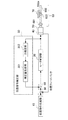•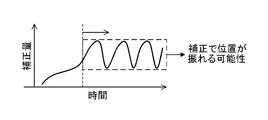•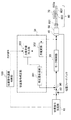•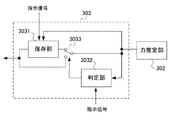•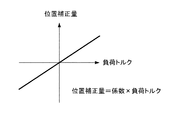•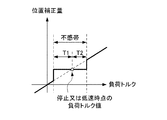•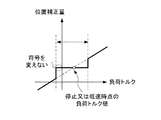•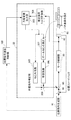••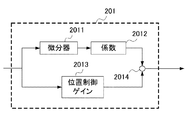••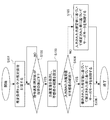•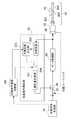••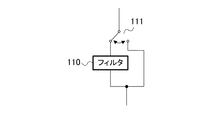•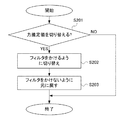•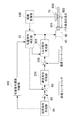•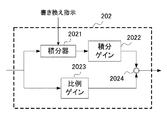•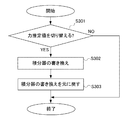## Classifications

• GPHYSICS
• G05CONTROLLING; REGULATING
• G05BCONTROL OR REGULATING SYSTEMS IN GENERAL; FUNCTIONAL ELEMENTS OF SUCH SYSTEMS; MONITORING OR TESTING ARRANGEMENTS FOR SUCH SYSTEMS OR ELEMENTS
• G05B19/00Programme-control systems
• G05B19/02Programme-control systems electric
• G05B19/04Programme control other than numerical control, i.e. in sequence controllers or logic controllers
• G05B19/042Programme control other than numerical control, i.e. in sequence controllers or logic controllers using digital processors
• G05B19/0423Input/output
• GPHYSICS
• G05CONTROLLING; REGULATING
• G05BCONTROL OR REGULATING SYSTEMS IN GENERAL; FUNCTIONAL ELEMENTS OF SUCH SYSTEMS; MONITORING OR TESTING ARRANGEMENTS FOR SUCH SYSTEMS OR ELEMENTS
• G05B19/00Programme-control systems
• G05B19/02Programme-control systems electric
• G05B19/18Numerical control [NC], i.e. automatically operating machines, in particular machine tools, e.g. in a manufacturing environment, so as to execute positioning, movement or co-ordinated operations by means of programme data in numerical form
• G05B19/19Numerical control [NC], i.e. automatically operating machines, in particular machine tools, e.g. in a manufacturing environment, so as to execute positioning, movement or co-ordinated operations by means of programme data in numerical form characterised by positioning or contouring control systems, e.g. to control position from one programmed point to another or to control movement along a programmed continuous path
• G05B19/195Controlling the position of several slides on one axis
• GPHYSICS
• G05CONTROLLING; REGULATING
• G05BCONTROL OR REGULATING SYSTEMS IN GENERAL; FUNCTIONAL ELEMENTS OF SUCH SYSTEMS; MONITORING OR TESTING ARRANGEMENTS FOR SUCH SYSTEMS OR ELEMENTS
• G05B11/00Automatic controllers
• G05B11/01Automatic controllers electric
• G05B11/011Automatic controllers electric details of the correcting means
• GPHYSICS
• G05CONTROLLING; REGULATING
• G05BCONTROL OR REGULATING SYSTEMS IN GENERAL; FUNCTIONAL ELEMENTS OF SUCH SYSTEMS; MONITORING OR TESTING ARRANGEMENTS FOR SUCH SYSTEMS OR ELEMENTS
• G05B13/00Adaptive control systems, i.e. systems automatically adjusting themselves to have a performance which is optimum according to some preassigned criterion
• G05B13/02Adaptive control systems, i.e. systems automatically adjusting themselves to have a performance which is optimum according to some preassigned criterion electric
• G05B13/0205Adaptive control systems, i.e. systems automatically adjusting themselves to have a performance which is optimum according to some preassigned criterion electric not using a model or a simulator of the controlled system
• G05B13/024Adaptive control systems, i.e. systems automatically adjusting themselves to have a performance which is optimum according to some preassigned criterion electric not using a model or a simulator of the controlled system in which a parameter or coefficient is automatically adjusted to optimise the performance
• GPHYSICS
• G05CONTROLLING; REGULATING
• G05BCONTROL OR REGULATING SYSTEMS IN GENERAL; FUNCTIONAL ELEMENTS OF SUCH SYSTEMS; MONITORING OR TESTING ARRANGEMENTS FOR SUCH SYSTEMS OR ELEMENTS
• G05B19/00Programme-control systems
• G05B19/02Programme-control systems electric
• G05B19/18Numerical control [NC], i.e. automatically operating machines, in particular machine tools, e.g. in a manufacturing environment, so as to execute positioning, movement or co-ordinated operations by means of programme data in numerical form
• G05B19/19Numerical control [NC], i.e. automatically operating machines, in particular machine tools, e.g. in a manufacturing environment, so as to execute positioning, movement or co-ordinated operations by means of programme data in numerical form characterised by positioning or contouring control systems, e.g. to control position from one programmed point to another or to control movement along a programmed continuous path
• GPHYSICS
• G05CONTROLLING; REGULATING
• G05BCONTROL OR REGULATING SYSTEMS IN GENERAL; FUNCTIONAL ELEMENTS OF SUCH SYSTEMS; MONITORING OR TESTING ARRANGEMENTS FOR SUCH SYSTEMS OR ELEMENTS
• G05B19/00Programme-control systems
• G05B19/02Programme-control systems electric
• G05B19/18Numerical control [NC], i.e. automatically operating machines, in particular machine tools, e.g. in a manufacturing environment, so as to execute positioning, movement or co-ordinated operations by means of programme data in numerical form
• G05B19/404Numerical control [NC], i.e. automatically operating machines, in particular machine tools, e.g. in a manufacturing environment, so as to execute positioning, movement or co-ordinated operations by means of programme data in numerical form characterised by control arrangements for compensation, e.g. for backlash, overshoot, tool offset, tool wear, temperature, machine construction errors, load, inertia
• GPHYSICS
• G05CONTROLLING; REGULATING
• G05BCONTROL OR REGULATING SYSTEMS IN GENERAL; FUNCTIONAL ELEMENTS OF SUCH SYSTEMS; MONITORING OR TESTING ARRANGEMENTS FOR SUCH SYSTEMS OR ELEMENTS
• G05B2219/00Program-control systems
• G05B2219/20Pc systems
• G05B2219/25Pc structure of the system
• G05B2219/25257Microcontroller
• GPHYSICS
• G05CONTROLLING; REGULATING
• G05BCONTROL OR REGULATING SYSTEMS IN GENERAL; FUNCTIONAL ELEMENTS OF SUCH SYSTEMS; MONITORING OR TESTING ARRANGEMENTS FOR SUCH SYSTEMS OR ELEMENTS
• G05B2219/00Program-control systems
• G05B2219/30Nc systems
• G05B2219/41Servomotor, servo controller till figures
• G05B2219/41092References, calibration positions for correction of value position counter
• GPHYSICS
• G05CONTROLLING; REGULATING
• G05BCONTROL OR REGULATING SYSTEMS IN GENERAL; FUNCTIONAL ELEMENTS OF SUCH SYSTEMS; MONITORING OR TESTING ARRANGEMENTS FOR SUCH SYSTEMS OR ELEMENTS
• G05B2219/00Program-control systems
• G05B2219/30Nc systems
• G05B2219/41Servomotor, servo controller till figures
• G05B2219/41138Torque compensation
• GPHYSICS
• G05CONTROLLING; REGULATING
• G05BCONTROL OR REGULATING SYSTEMS IN GENERAL; FUNCTIONAL ELEMENTS OF SUCH SYSTEMS; MONITORING OR TESTING ARRANGEMENTS FOR SUCH SYSTEMS OR ELEMENTS
• G05B2219/00Program-control systems
• G05B2219/30Nc systems
• G05B2219/41Servomotor, servo controller till figures
• G05B2219/41164How to compensate, for example by injecting compensation signal in comparator of normal loop

## Description

The present invention relates to a servo motor control device, a servo motor control method, and a servo motor control program having a function of correcting the position of a driven body driven by the power of a servo motor.
2. Description of the Related Art Conventionally, there is a servo motor control device in which a non-workpiece (work) is mounted on a table and the table is moved by a servo motor via a coupling mechanism. The table and work are driven bodies. The coupling mechanism has a coupling coupled to the motor servo and a ball screw fixed to the coupling. The ball screw is screwed onto the nut.
Among such servo motor control apparatuses, there is a servo motor control apparatus having a function of correcting the position of a driven body (also referred to as a moving body) driven by the power of the servo motor.
For example, Patent Document 1 describes that a driving force acting on a driven body is estimated at a connecting portion of a connecting mechanism, and a position command value is corrected based on the estimated driving force.
In Patent Document 2, the amount of expansion and contraction of the ball screw is calculated from the distance from the servo motor to the moving body and the torque command value, and the position correction amount of the moving body screwed to the ball screw is calculated from the amount of expansion and contraction. There is a description that the position command value is corrected by the position correction amount.
Further, in Patent Document 3, a servo control device includes a ball screw, a tension acting on the distal side from the servo motor, a distance between a pair of fixed portions that support the ball screw at both ends, and a servo motor. The amount of expansion and contraction of the ball screw is calculated based on the distance from the fixed part provided on the proximal side to the moving body and the torque command given to the servo motor, and the feed based on the calculated amount of expansion and contraction of the ball screw. There is a description of calculating an axis position correction amount.
JP 2014-109785 A JP 2014-13554 A JP 2014-87880 A
When correcting the position command value, the inventors add a correction unrelated to the machine operation to the position command value in response to the estimated driving force at the time of stop and low speed operation, and the correction amount fluctuates. I found it to happen.
An object of the present invention is to provide a servo motor control device, a servo motor control method, and a servo motor control program capable of controlling the position of a driven body with higher accuracy in machine tools and industrial machines.
(1) A servo motor control device according to the present invention includes a servo motor (for example, a servo motor 50 described later),
A driven body (for example, a table 70 described later) driven by the servo motor;
A coupling mechanism (for example, a coupling 601 and a ball screw 602 described later) that couples the servo motor and the driven body and transmits the power of the servo motor to the driven body;
A position command generation unit (for example, a position command generation unit 10 described later) that generates a position command value of the driven body;
A motor control unit (for example, a motor control unit 20 described later) that controls the servo motor using the position command value;
A force estimation unit (e.g., a force estimation unit 302 described later) that estimates a force estimation value that is a driving force acting on the driven body at a coupling unit with the coupling mechanism;
Based on the position command value, the reflection of the update of the force estimation value is determined to be reflected and the update is stopped, the force estimation value reflecting the update, and the force estimation value when the update is stopped based on the position command value A force estimated value output unit (for example, a force estimated value output unit 303 described later),
A correction amount generation unit (for example, a correction amount generation unit 301 described later) that generates a correction amount to correct the position command value using the output of the force estimated value output unit;
Is a servo motor control device.
(2) In the servo motor control device according to (1), the force estimated value output unit is configured such that when the command speed of the position command value or the command acceleration of the position command value is a predetermined value or less, It may be determined whether to stop updating the force estimation value estimated by the force estimation unit.
(3) In the servo control device according to (1) or (2), the force estimated value output unit is configured such that the command speed of the position command value or the command acceleration of the position command value is a predetermined value or less. In addition, a predetermined force estimated value range is defined with reference to the force estimated value when the update is stopped, and if the force estimated value output from the force estimating unit is within the force estimated value range, the update is performed. You may output the force command value at the time of stopping.
(4) In the servo control device according to any one of (1) to (3) above, the correction amount generation unit is configured to calculate the force estimated value output from the force estimated value output unit and the first coefficient. The sum of the product, the product of the estimated force output from the estimated force output unit, the distance from the servo motor to the connecting unit, and the second coefficient may be used as the correction amount.
(5) In the servo control device according to (3), the force estimated value ranges are different force estimated value ranges with respect to the magnitude direction of the force estimated value with reference to the force estimated value when the update is stopped. May be provided.
(6) In the servo control device according to any one of (1) to (5), when the command speed or the command acceleration of the position command value generated by the position command generation unit exceeds a predetermined value, the force estimation A switching unit (for example, a switch 304 described later) for switching from the force estimation value output from the value output unit to the force estimation value estimated by the force estimation unit may be provided.
(7) In the servo control device according to any one of (1) to (6), the force estimated value output unit outputs the force estimated value output based on the force estimated value reflecting the update and the position command value. When switching between the estimated force value when the update is stopped or when the switching unit outputs the estimated force value output from the estimated force value output unit from the estimated force value output unit and the estimated force unit When switching between the estimated force values to be output, the correction amount generated by the correction amount generation unit may be filtered (for example, a filter 110 described later).
(8) In the servo control device according to any one of (1) to (7), the motor control unit includes a speed command generating unit (for example, a speed command generating unit 201 described later) and a torque command generating unit (for example, described later). Torque command generating unit 202),
The torque command generating unit has an integrator (for example, an integrator 2021 described later) that integrates at least the speed deviation,
When the force estimated value output unit switches the output force estimated value between the force estimated value reflecting the update and the force estimated value when the update is stopped based on the position command value, or the switching The integrator rewrites when the force estimation value to be output is switched between the force estimation value output from the force estimation value output unit and the force estimation value output from the force estimation unit. May be performed.
(9) The servo motor control method of the servo motor control device of the present invention includes a servo motor (for example, a servo motor 50 described later),
A driven body (for example, a table 70 described later) driven by the servo motor;
A servo motor control device comprising: a coupling mechanism (for example, a coupling 601 and a ball screw 602 described later) that couples the servo motor and the driven body and transmits the power of the servo motor to the driven body. In the servo motor control method,
Generating a position command value of the driven body;
Estimating a force estimation value that is a driving force acting on the driven body at a connecting portion with the connecting mechanism;
Based on the position command value, the reflection of the update of the force estimation value is determined to be reflected and the update is stopped, the force estimation value reflecting the update, and the force estimation value when the update is stopped based on the position command value And correcting the generated position command value based on the selected force estimation value,
This is a servo motor control method for controlling the servo motor using the corrected position command value.
(10) The servo motor control program of the present invention includes a servo motor (for example, a servo motor 50 described later),
A driven body (for example, a table 70 described later) driven by the servo motor;
A servo motor control device comprising: a coupling mechanism (for example, a coupling 601 and a ball screw 602 described later) that couples the servo motor and the driven body and transmits the power of the servo motor to the driven body. A servo motor control program for causing a computer to execute servo motor control,
In the computer,

Processing for generating a position command value of the driven body;
A process of estimating a force estimation value which is a driving force acting on the driven body at a connecting portion with the connecting mechanism;
Based on the position command value, the reflection of the update of the force estimation value is determined to be reflected and the update is stopped, the force estimation value reflecting the update, and the force estimation value when the update is stopped based on the position command value A process of selecting one of and
Processing for correcting the generated position command value based on the selected force estimation value;
A process of controlling the servo motor using the corrected position command value;
This is a servo motor control program that executes.
According to the present invention, even when the vehicle is stopped or operated at a low speed, the fluctuation of the correction amount caused by the addition of the correction not related to the machine operation to the position command value in response to the estimated driving force is suppressed, and the accuracy is increased. The position of the driving body can be controlled.
It is a block diagram which shows the structure of the servomotor control apparatus used as a premise technique. It is a figure explaining the fluctuation | variation of a correction amount. It is a block diagram which shows the structure of the servomotor control apparatus used as the 1st Embodiment of this invention. It is a block diagram which shows the structure of the force estimated value output part of FIG. It is a characteristic view which shows operation | movement of the servomotor control apparatus shown in FIG. It is a characteristic view which shows operation | movement of the servomotor control apparatus shown in FIG. It is a characteristic view which shows operation | movement of the servomotor control apparatus shown in FIG. It is a block diagram which shows the structure of the servomotor control apparatus containing one structural example of a position command correction | amendment part. It is a block diagram which shows the example of 1 structure of a motor control part, and the structure of the servomotor control apparatus containing the distance calculation part which calculates | requires the length (length of a spring element) of a ball screw. It is a block diagram which shows one structural example of a speed instruction | command preparation part. It is a block diagram which shows the example of 1 structure of a torque instruction | command preparation part. It is a flowchart which shows operation | movement of the servomotor control apparatus shown in FIG. It is a block diagram which shows the structure of the servomotor control apparatus used as the 2nd Embodiment of this invention. It is a block diagram which shows the structure of the servomotor control apparatus used as the 3rd Embodiment of this invention. It is a figure which shows a filter and the changeover switch of a filter. It is a flowchart which shows the switching operation | movement of the filter by a change switch. It is a block diagram which shows the structure of the servomotor control apparatus used as the 4th Embodiment of this invention. It is a block diagram which shows the structure of a torque instruction | command preparation part. It is a flowchart which shows the switching operation | movement of an integrator.
Hereinafter, embodiments of the present invention will be described with reference to the drawings.
First, prior to the description of the embodiment of the present invention, a servo motor control device as a prerequisite technology will be described.
FIG. 1 is a block diagram showing a configuration of a servo motor control device as a prerequisite technology. The servo motor control device moves the table 70 via the coupling mechanism 60 by the servo motor 50 to process a workpiece (workpiece) mounted on the table 70. The coupling mechanism 60 has a coupling 601 coupled to the servo motor 50 and a ball screw 602 fixed to the coupling 601, and a nut 603 is screwed to the ball screw 602. The nut 603 screwed to the ball screw 602 moves in the axial direction of the ball screw 602 by the rotational drive of the servo motor 50.
The rotation angle position of the servo motor 50 is detected by an encoder 40 that is associated with the servo motor 50 and serves as a position detection unit, and the detected position (position detection value) is used as position feedback. The encoder 40 can detect the rotation speed, and the detected speed (speed detection value) can be used as speed feedback.
The servo motor control device is created by a position command generation unit 10 that generates a position command value of the servo motor 50 and a position command generation unit 10 according to a program or command input from a host control device or an external input device (not shown). Using a subtractor 80 for obtaining a difference between the position command value and the position detection value detected by the encoder 40, an adder 90 for adding the difference and a correction value output from the position command correction unit 30, and using this addition value. And a motor control unit 20 that creates a torque command value for the servo motor 50.
When the servo motor 50 is driven, a driving force acts on the coupling mechanism 60 and the table 70, and these are elastically deformed. However, since the coupling mechanism 60 has lower rigidity than the table 70, of the entire elastic deformation, The elastic deformation of the coupling mechanism 60 accounts for the majority. When the coupling mechanism 60 is elastically deformed, an error corresponding to the amount of elastic deformation is generated at the position of the table 70 even when the servo motor 50 is rotated according to the command value. Therefore, in order to eliminate this error, the position command value is corrected by the amount of elastic deformation of the coupling mechanism 60. The amount of elastic deformation of the connecting mechanism 60 is proportional to the driving force acting on the table 70 at the connecting portion 603a between the table 70 (nut 603) and the connecting mechanism 60, and the driving force can be expressed by the driving torque acting on the connecting portion. it can.
The position command correction unit 30 includes a correction amount generation unit 301 and a force estimation unit 302. The force estimation unit 302 estimates a driving force (driving torque) acting on the driving body at the connecting unit using the torque command value. The correction amount generation unit 301 generates a correction amount for correcting the position command value generated by the position command generation unit 10 based on the driving force estimated by the force estimation unit 302 and outputs a correction value.
The servo motor control device of the base technology shown in FIG. 1 adds a correction not related to the mechanical operation to the position command value in response to the estimated driving force even during stop and low speed operation, As shown in FIG. 2, it has been found that there is a case where the correction amount fluctuates. The present inventors have found that the fluctuation of the correction amount can be suppressed if the update of the force estimation value output from the force estimation unit is stopped during the stop or low speed operation.
Hereinafter, an embodiment of a servo motor control device of the present invention that suppresses fluctuation of the correction amount will be described. The machine to which the servo motor control device of the present embodiment described below is applied is a machine tool such as a laser beam machine, an electric discharge machine, a cutting machine, etc., but the servo motor control device of the present invention is an industry such as a robot. It can also be applied to machines.
(First embodiment)
FIG. 3 is a block diagram showing the configuration of the servo motor control apparatus according to the first embodiment of the present invention. FIG. 4 is a diagram illustrating a configuration example of the force estimation value output unit. In FIG. 3, the same components as those of the servo motor control device of FIG.
As shown in FIG. 3, in this embodiment, the position command correction unit 31 includes a position command speed determination unit 100 and a force estimated value output unit 303 compared to the position command correction unit 30 shown in FIG. 1. . As shown in FIG. 4, the force estimated value output unit 303 includes a storage unit 3031, a determination unit 3032, and a switch 3033.
The position command speed determination unit 100 receives the position command value created by the position command generation unit 10, generates a command speed (position command speed) of the position command value, and the command speed (absolute value) is equal to or less than a predetermined value ( 0 is included), and an instruction signal is sent to the storage unit 3031 and the determination unit 3032 of the force estimation value output unit 303. When the command speed (absolute value) is equal to or less than a predetermined value (including 0), it is determined that the servo motor control device is performing control at a stop or at a low speed. Here, the position command speed determination unit 100 determines whether or not the command speed (absolute value) is equal to or less than a predetermined value (including 0), but the position command speed determination unit 100 only generates the command speed. The determination unit 3032 may determine whether the command speed (absolute value) is equal to or less than a predetermined value (including 0) based on the command speed received from the position command speed determination unit 100.
Based on the instruction signal, the force estimated value output unit 303 determines whether the update of the force estimated value from the force estimating unit 302 is reflected and stops updating, and the force estimated value reflecting the update (from the force estimating unit 302). Force estimation value) or a force estimation value when the update is stopped based on the instruction signal (force estimation value stored in the storage unit 3031) is output.
When the storage unit 3031 of the force estimation value output unit 303 receives the instruction signal from the position command speed determination unit 100, when the instruction signal indicates that the command speed (absolute value) is equal to or less than a predetermined value, the force estimation The estimated force value (estimated load torque) from the unit 302 is stored. The estimated force value to be stored corresponds to the estimated force value at the time of stop or low speed.
The determination unit 3032 receives the instruction signal from the position command speed determination unit 100, and when the instruction signal indicates that the command speed (absolute value) is equal to or less than a predetermined value, the force estimation value (from the force estimation unit 302) A predetermined force estimation value range (a predetermined dead zone range) is set with reference to a force estimation value that is substantially the same as the force estimation value stored in the storage unit 3031. Thereafter, the determination unit 3032 determines whether or not the force estimation value input next from the force estimation unit 302 is within a predetermined force estimation value range. If the force estimation value is within the predetermined force estimation value range, the determination unit 3032 switches the switch 3033 so that the input side of the storage unit 3031 and the output side of the force estimation unit 302 are connected, and outputs the result to the storage unit 3031. An instruction signal is output. Receiving the output instruction signal, the storage unit 3031 outputs the stored force estimation value to the correction amount generation unit 301.
On the other hand, if the input force estimation value is not within the predetermined force estimation value range, the determination unit 3032 sets the switch 3033 so that the input side of the correction amount generation unit 301 and the output side of the force estimation unit 302 are connected. Switching is performed, and the input force estimation value is output to the correction amount generation unit 301. When the instruction signal indicates that the command speed (absolute value) is not equal to or less than the predetermined value (the command speed (absolute value) exceeds the predetermined value), the determination unit 3032 causes the switch 3033 to generate a correction amount. It switches so that the input side of the part 301 and the output side of the force estimation part 302 may be connected.
In this way, the correction amount generation unit 301 stores the force estimation value (estimated load torque) from the force estimation unit 302 in the storage unit 3031 when the force estimation value (estimated load torque) is within a predetermined force estimation value range (in the dead zone). A correction amount for correcting elastic deformation is generated using the estimated force value thus estimated as an estimated load torque, and is output to the adder 90. do. On the other hand, when the force estimation value (estimated load torque) from the force estimation unit 302 is outside the predetermined force estimation value range, the correction amount generation unit 301 acquires the force estimation value acquired in real time from the force estimation unit 302. As an estimated load torque, a correction amount for correcting elastic deformation is generated and output to the adder 90.
The command acceleration of the position command value may be used instead of the command speed of the position command value. In this case, the position command speed determination unit 100 receives the position command value created by the position command generation unit 10 and receives the position command value. It is replaced with a position command acceleration determination unit that generates a command acceleration (position command acceleration) of the command value. The function of the position command acceleration determination unit is the same as that of the position command speed determination unit 100 except that the command speed is replaced with the command acceleration. Here, the switch 304 is switched based on the position command speed or the position command acceleration. However, the position command speed or the position command acceleration may not be used if a stop and low speed operation can be detected based on the position command.
As already described, the force estimating unit 302 estimates and outputs the driving force (driving torque) acting on the driving body at the connecting unit using the torque command value output from the motor control unit 20. This estimated load torque value is the estimated force value. The estimation of the driving force is not limited to this. For example, as described in Patent Document 1 (Japanese Patent Laid-Open No. 2014-109785), the driving force is estimated by further adding acceleration / deceleration torque, disturbance torque, or the like. The driving force may be estimated by calculating the motor torque using the output of the current detection unit that detects the motor current instead of the torque command value.
Hereinafter, the operation of the servo motor control apparatus of this embodiment will be described with reference to the characteristic diagrams of FIGS. 5, 6, and 7.
In the servo motor control device shown in FIG. 1, when the influence of the length of the ball screw is not taken into consideration, the load torque and the correction amount are in a proportional relationship as shown in FIG. 5, and the position correction amount = coefficient × load torque Therefore, when the load torque changes when the command is stopped, the position correction amount also changes.
In this embodiment, as shown in FIG. 6, when the command speed or command acceleration of the position command value becomes equal to or less than a predetermined value, the load torque value (becomes an estimated force value) when the value becomes equal to or less than the predetermined value. That is, a predetermined dead zone based on the load torque during stoppage or at low speed is provided for the load torque output from the force estimation unit 302. In this dead zone, the correction amount generation unit 301 performs correction based on the estimated value (load torque) during stoppage or low speed output from the storage unit 3031 of the force estimation value output unit 303. This makes it possible to prevent an unnecessary change in the correction amount due to a minute change in the load torque. When a change exceeding the predetermined dead zone width occurs in the load torque output from the force estimation unit 302, the correction amount generation unit 301 performs correction based on the estimated force value output from the force estimation unit 302.
When the correction amount generation unit 301 performs correction based on the estimated force value output from the force estimation unit 302, if the load torque falls within the dead band again, the load during stoppage or low speed A predetermined dead zone is provided for the load torque output from the force estimation unit 302 based on the torque value. This is repeated.
The width of the dead zone may not be uniform in the magnitude direction from the load torque during stoppage or at low speed. That is, as shown in FIG. 6, the widths T1 and T2 in the magnitude direction from the load torque during stoppage or low speed are T1 = T2, T1> T2 or T1 <T2. Good. The sign may change (for example, the load torque changes from positive to negative) due to the influence of the load torque at the time of stopping or at low speed. Even in this case, as shown in FIG. The sign of the estimated load torque at the time is not changed.
As described above, in the present embodiment, a predetermined force estimated value range (a predetermined dead zone range) is set when the motor is stopped or at a low speed with respect to the estimated load torque for calculating the elastic deformation of a machine such as a ball screw. If the estimated force value from the force estimating unit 302 is within the predetermined estimated force value range, the updating of the load torque is stopped. The correction amount generation unit 301 generates a correction amount using a predetermined force estimated value (force estimated value stored in the storage unit) for which updating has been stopped, and avoids excessive correction.
According to the present embodiment, only correction for lost motion can be performed without reflecting a minute change in correction amount during stoppage or at low speed that is not related to the operation of the servomotor.
FIG. 8 is a block diagram showing a configuration of a servo motor control apparatus including a configuration example of the position command correction unit. An estimated load torque calculation unit 308 of the position command correction unit 32 in FIG. 8 corresponds to the force estimation unit 302 in FIG. 3 and includes a torsion coefficient multiplication unit 305, a ball screw length multiplication unit 307, a shape factor multiplication unit 306, The addition unit 309 corresponds to the correction amount generation unit 301 in FIG. The shape factor indicates the amount of expansion / contraction per unit length of the ball screw. In the present embodiment, the torsional elastic deformation around the rotating shaft and the elastic elastic deformation in the axial direction that occur in the coupling mechanism (coupling, ball screw) are calculated based on the estimated load torque or fixed value, resulting from the elastic deformation. Correct the lost motion with respect to the position command value. In this example, a force estimated value output unit is provided on the input side of the ball screw length multiplying unit 307 and processing for stopping the update of the force estimated value is performed, so that the position correction amount depends on the length of the ball screw. I can make you wait. At this time, the elastic deformation in the axial direction depends on the distance from the servo motor to the driven body, and this distance is estimated from the integrated value of the movement position.
If the estimated load torque is T and the torsion coefficient is α, the correction amount relating to the torsion of the connecting portion is α · T. If the estimated load torque is T, the length of the ball screw is d, and the shape factor is β, the expansion and contraction of the ball screw is The correction amount relating to is d · β · T. The total correction amount obtained by adding these corrections by the adding unit 309 is α · T + d · β · T. The length d of the ball screw is the length of the ball screw from the servo motor to the connecting portion, and varies depending on the position of the table.
FIG. 9 is a block diagram showing a configuration example of the motor control unit 20 and a configuration of a servo motor control device including a distance calculation unit 130 for obtaining the length of the ball screw (the length of the spring element). The distance of the ball screw multiplied by the ball screw length multiplication unit 307 in FIG. 8 (the length of the spring element) is calculated by the distance calculation unit 130. The motor control unit 20 in FIG. 8 includes a speed command creation unit 201, a subtraction unit, and a torque command creation unit 202.
FIG. 10 is a block diagram illustrating a configuration example of the speed command creation unit 201. As shown in FIG. 8, the position command generation unit 10 creates a position command value, obtains a difference between the position command value and the detected position fed back by the subtractor 80, and adds a correction amount to the difference by the adder 90. In addition, the difference obtained by adding the correction amount is input to a differentiator 2011 and a position control gain 2013 shown in FIG. The adder 2014 outputs an addition value of the output of the coefficient unit 2012 obtained by multiplying the output of the differentiator 2011 by a coefficient and the output of the position control gain 2013 as a speed command value. The subtractor 120 obtains the difference between the speed command value and the detected speed that is speed-feedback.
FIG. 11 is a block diagram illustrating a configuration example of the torque command creating unit 202. The torque command generator 202 adds the output of the proportional gain 2023 connected to the subtractor 120, the integrator 2021, the integral gain 2022 connected to the integrator 2021, and the proportional gain 2023 and the output of the integral gain 2022. An adder 2024 that outputs to the servo motor 50 as a torque command is provided. The integrator 2021 integrates the input. The integral gain 2022 multiplies the output of the integrator 2021 by a coefficient, and the proportional gain 2023 multiplies the input by a coefficient. The order of integration gain 2022 and integrator 2021 may be changed.
FIG. 12 is a flowchart showing the operation of the servo motor control apparatus shown in FIG. In step S101, the force estimation unit 302 calculates an estimated load torque (force estimation value). In step S102, the position command speed determination unit determines whether or not the generated command speed (absolute value) is equal to or less than a predetermined value, and determines that the command speed (absolute value) is equal to or less than the predetermined value (step S102). In the case of YES), an instruction signal is sent to the force estimated value output unit 303. The storage unit 3031 of the force estimated value output unit 303 stores the force estimated value when the instruction signal is received, and the determination unit 3032 sets the dead zone range based on the force estimated value when the instruction signal is received. (Step S103). On the other hand, if the position command speed determination unit determines that the command speed (absolute value) cannot be equal to or less than a predetermined value (NO in step S102), the process returns to step S102.
In step S <b> 104, the determination unit 3032 determines whether the force estimation value input next from the force estimation unit 302 is within the set dead band range. When the input force estimation value is within the set dead band range (YES in step S104), the determination unit 3032 switches the switch 3033 and outputs an output instruction signal to the storage unit 3031. In step S106, the correction amount generation unit 301 generates a position correction amount based on the load torque stored in the storage unit 3031 and calculates the difference between the position command value from the position command generation unit 10 and the position feedback detected position. The correction is performed using the position correction amount calculated by the correction amount generation unit 301. Then, the servo motor 50 is controlled by the motor control unit 20 based on the corrected difference between the position command value and the detected position.
On the other hand, when the input force estimation value is not within the set dead band range (NO in step S104), the determination unit 3032 switches the switch 3033 to change the force estimation value from the force estimation unit 302 to the correction amount. The data is output to the generation unit 301. In step S105, the correction amount generation unit 301 generates a position correction amount based on the force estimation value from the force estimation unit 302, and calculates the difference between the position command value from the position command generation unit 10 and the position feedback detection position. The correction is performed using the position correction amount calculated by the correction amount generation unit 301. Then, the servo motor 50 is controlled by the motor control unit 20 based on the corrected difference between the position command value and the detected position.
(Second Embodiment)
FIG. 13 is a block diagram showing a configuration of a servo motor control apparatus according to the second embodiment of the present invention.
As shown in FIG. 13, in the servo motor control device of the present embodiment, the position command correction unit 32 includes a switch 304 serving as a switching unit added to the position command correction unit 31 of the servo motor control device shown in FIG. 3. The switch 304 is switched by a switching signal from the position command speed determination unit 100.
When the command speed (absolute value) is equal to or less than a predetermined value (including 0), the position command speed determination unit 100 is configured such that the input end of the correction amount generation unit 301 is connected to the output end of the force estimation value output unit 303. A switching signal is sent to the switch 304. The position command speed determination unit 100 switches the switching signal so that the input end of the correction amount generation unit 301 is connected to the output end of the force estimation unit 302 when the command speed (absolute value) exceeds a predetermined value. 304. The command acceleration of the position command value may be used instead of the command speed of the position command value. In this case, the position command speed determination unit 100 receives the position command value created by the position command generation unit 10 and receives the position command value. It is replaced with a position command acceleration judgment unit that generates a command acceleration of value (position command acceleration). The function of the position command acceleration determination unit is the same as that of the position command speed determination unit 100 except that the command speed is replaced with the command acceleration.
In the present embodiment, when the command speed (absolute value) exceeds a predetermined value, the switch 304 switches so that the input end of the correction amount generation unit 301 is connected to the output end of the force estimation unit 302. Therefore, as in the first embodiment, the determination unit can quickly perform the switching operation without determining whether or not the force estimation value is within the predetermined force estimation value range.
Although the switch 304 is provided in the present embodiment, when the command speed (absolute value) exceeds a predetermined value, the determination unit 3032 in FIG. 4 switches the switch 3033 so that the input side of the correction amount generation unit 301 and the force estimation unit If the output side of 302 is connected, the switch 304 need not be provided.
(Third embodiment)
In the servo motor control device of the first embodiment, the determination unit 3032 determines the force from the force estimation value stored in the storage unit 3031 from the force estimation value from the force estimation unit 302 or the force estimation value stored in the storage unit 3031. When switching to the force estimation value from the estimation unit 302, the correction amount may change discontinuously at the time of switching. The discontinuous correction amount becomes a discontinuous speed command, and generates a discontinuous torque command. In order to prevent this correction amount discontinuity, a filter 110 is provided at the output of the position command correction unit 31. By passing the correction amount through the low-pass filter, the discontinuous value can be gently followed.
FIG. 14 is a block diagram showing a configuration of a servo motor control apparatus according to the third embodiment of the present invention. The configuration of the servo motor control device of FIG. 14 is the same as the configuration of the servo motor control device of FIG. 3 except that the filter 110 is provided, and the same components are denoted by the same reference numerals and description thereof is omitted. To do. A low-pass filter can be used as the filter 110.
As described above, by connecting the filter 110 between the correction amount generation unit 301 and the adder 90, it is possible to prevent the correction amount from being discontinuous. However, the changeover switch 111 shown in FIG. 15 may switch between the case where the filter 110 is not used and the case where the filter 110 is used. The determination unit 3032 uses the switch 3033 to calculate the force estimation value stored in the storage unit 3031 from the force estimation value from the force estimation unit 302 or the force estimation value stored in the storage unit 3031 from the force estimation unit 302. Only when switching to the force estimation value, the changeover switch 111 connects the filter 110 between the correction amount generator 301 and the adder 90. After the switching by the switch 3033, the changeover switch 111 connects the correction amount generation unit 301 and the adder 90 so as not to pass through the filter 110. The changeover switch 111 is switched based on an instruction signal from the determination unit 3022 shown in FIG.
FIG. 16 is a flowchart showing the switching operation of the filter 110 by the changeover switch 111. In step S201, the determination unit 3032 determines whether to switch the force estimation value. When the force estimation value is switched (YES in step S201), the switch 111 is switched to apply a filter in step 202, Thereafter, when the discontinuity of the correction amount is resolved, the changeover switch 111 is switched so as not to apply the filter in step 203. When the determination unit 3032 switches the force estimation value, the force estimation value from the force estimation unit 302 is switched to the force estimation value stored in the storage unit 3031, and the force estimation is performed from the force estimation value stored in the storage unit 3031. There is a case where the force estimated value from the unit 302 is switched to.
In the above description, an example in which a filter or a filter and a changeover switch are added to the configuration of the servo motor control device in FIG. 3 has been described. However, a filter or a filter and a changeover switch are added to the configuration of the servo motor control device in FIG. May be. In this case, there are two cases in which a filter is provided. In the first case, the determination unit 3032 uses the switch 3033 to estimate the force estimation value stored in the storage unit 3031 from the force estimation value from the force estimation unit 302 or the force estimation value stored in the storage unit 3031. Is switched to the estimated force value from the force estimation unit 302. In the second case, the position command speed determination unit 100 uses the switch 304 to determine the force estimation value from the force estimation value output unit 303, the force estimation value from the force estimation unit 302, or the force estimation unit 302 from This is a case of switching from the force estimated value to the force estimated value from the force estimated value output unit 303.
When a filter and a changeover switch are added, the changeover switch 111 is switched based on an instruction signal from the determination unit 3032 or the position command speed determination unit 100 shown in FIG. The position command speed determination unit 100 may be replaced with a position command acceleration determination unit that receives a position command value created by the position command generation unit 10 and generates a command acceleration (position command acceleration) of the position command value.
(Fourth embodiment)
In the servo motor control apparatus of the third embodiment, the filter 110 is provided at the output of the position command correction unit 31 in order to prevent discontinuity of the correction amount, but instead of the filter, the torque command generation unit 202 shown in FIG. The integrator 2021 may be rewritten so that the torque command value becomes continuous. In the present embodiment, the filter 110 may be provided at the output of the position command correction unit 31 together with the rewriting of the integrator 2021. Using the switch 3033, the determination unit 3032 uses the force estimation value stored in the storage unit 3031 from the force estimation value from the force estimation unit 302 or the force from the force estimation unit 302 based on the force estimation value stored in the storage unit 3031. If a correction amount for correcting the position command value is entered when switching to the estimated value, a change in the speed command value occurs. The integrator is rewritten so that the torque command value does not change due to the change of the speed command value so that the torque command value becomes continuous.
FIG. 17 is a block diagram showing a configuration of a servo motor control apparatus according to the fourth embodiment of the present invention. The configuration of the servo motor control device in FIG. 17 is the same as the configuration of the servo motor control device in FIG. 9 except that the determination unit 3032 shown in FIG. 4 sends an integrator rewrite instruction to the torque command creation unit 202. The same constituent members are denoted by the same reference numerals and description thereof is omitted. FIG. 18 is a block diagram showing a configuration of the torque command creating unit 202. As shown in FIG. 18, a rewrite instruction is sent to the integrator 2021.
The rewriting instruction is received from the force estimation unit 302 based on the force estimation value stored in the storage unit 3031 from the force estimation value from the force estimation unit 302 or the force estimation value stored in the storage unit 3031 by the determination unit 3032 shown in FIG. Sent when switching to the estimated force value. The rewriting of the integrator 2021 is performed as follows based on the rewriting instruction.
The torque command value TCMD is expressed as follows: Vcmd is a speed command value, Vfb is a detected speed, kp is a proportional gain, and ki is an integral gain.
It is expressed.
When the update of the correction amount is restarted after the update of the correction amount is stopped, the torque command value TCMD is changed from TCMD (1) to TCMD (2).
In the present embodiment, TCMD (1) + (Vcmd (2) −Vfb (2)) of the torque command value TCMD (2) is rewritten as the following Equation 3.
Then, the rewritten torque command value TCMD ′ (2) becomes the same as TCMD (1), and the torque command is not discontinuous.
FIG. 19 is a flowchart showing the switching operation of the integrator 2021. In step S301, the determination unit 3032 determines whether to switch the force estimation value. When the force estimation value is switched (YES in step S301), the integrator is rewritten in step 302. Thereafter, when the discontinuity of the correction amount is resolved, the integrator rewrite is restored in step 303. When the determination unit 3032 switches the force estimation value, the force estimation value from the force estimation unit 302 is switched to the force estimation value stored in the storage unit 3031, and the force estimation is performed from the force estimation value stored in the storage unit 3031. There is a case where the force estimated value from the unit 302 is switched to.
The above description of the present embodiment is based on the configuration of the servo motor control device of FIG. 9, in which the determination unit 3032 in the estimated force output unit 303 of the position command correction unit 32 sends an instruction to rewrite the integrator to the torque command generation unit 202. However, the configuration of this embodiment can also be applied to the configuration of the servo motor control device of FIG. 9 in which the position command speed determination unit 100 and the switch 304 of the servo motor control device of FIG. 13 are added. In this case, there are two cases where an integrator rewrite instruction is sent. In the first case, the determination unit 3032 uses the switch 3033 to estimate the force estimation value stored in the storage unit 3031 from the force estimation value from the force estimation unit 302 or the force estimation value stored in the storage unit 3031. Is switched to the estimated force value from the force estimation unit 302. In the second case, the position command speed determination unit 100 uses the switch 304 to determine the force estimation value from the force estimation value output unit 303, the force estimation value from the force estimation unit 302, or the force estimation unit 302 from This is a case of switching from the force estimated value to the force estimated value from the force estimated value output unit 303.
The integrator rewrite instruction is performed based on an instruction signal from the determination unit 3032 or the position command speed determination unit 100 shown in FIG. The position command speed determination unit 100 may be replaced with a position command acceleration determination unit that receives a position command value created by the position command generation unit 10 and generates a command acceleration (position command acceleration) of the position command value.
Although the embodiments of the present invention have been described above, the servo motor control device can realize all or part of its functions by hardware, software, or a combination thereof. Here, “realized by software” means realized by a computer reading and executing a program. When configured by hardware, a part or all of the correction amount generation unit 301, the force estimation unit 302, the force estimation value output unit 303, the position command generation unit 10, and the motor control unit 20 of the servo motor control device are, for example, LSI (Large Scale Integrated circuit), ASIC (Application Specific Integrated Circuit), gate array, FPGA (Field Programmable Gate Array) and other integrated circuits (IC).
When realized by software, a part or all of the servo motor control device is configured by a computer including a CPU and a storage unit such as a hard disk and a ROM storing a program, and FIG. 3, FIG. 4, FIG. 12 and the program according to the flowchart of FIG. 12, the program according to the block diagram of FIG. 13, or the program according to the block diagrams of FIGS. 14 and 15 and the flowchart of FIG. 16, and the block diagrams of FIGS. In accordance with the program according to the flowchart of FIG. 19, information necessary for calculation is stored in a second storage unit such as a RAM, and the process is executed to program a part or all of the operation of the servo motor control device. Can be done with. The program can be read into a storage unit such as a hard disk from an external storage medium such as a CD-ROM, DVD, or flash memory in which the program is recorded.
DESCRIPTION OF SYMBOLS 10 Position command generation part 20 Motor control part 30, 31, 32 Position command correction | amendment part 40 Encoder 50 Servo motor 60 Connection mechanism 70 Table 100 Position command speed determination part 110 Filter 301 Correction amount generation part 302 Force estimation part 303 Force estimation value output Part 303
304 switch

## Claims (8)

1. A servo motor,
A driven body driven by the servo motor;
A coupling mechanism for coupling the servo motor and the driven body, and transmitting the power of the servo motor to the driven body;
A position command generator for generating a position command value of the driven body;
A motor control unit for controlling the servo motor using the position command value;
A force estimation unit that estimates a force estimation value that is a driving force acting on the driven body at a connection portion with the connection mechanism;
When the command speed of the position command value is equal to or less than a predetermined value, a predetermined force estimated value range is defined with reference to the force estimated value at that time, and the force estimated value output from the force estimating unit is If it is within the force estimation value range, it outputs a force command value for which updating of the force estimation value is stopped, and if the force estimation value output from the force estimation unit is outside the force estimation value range, the force A force estimated value output unit that outputs a force command value reflecting the update of the estimated value;
Using the output of the power estimation value output unit, and a correction quantity generating unit for generating a correction amount for correcting the position command value,
Servo motor control device comprising:
2. The correction amount generation unit includes a product of the force estimation value output from the force estimation value output unit and the first coefficient, the force estimation value output from the force estimation value output unit, and the servo motor. The servo motor control device according to claim 1, wherein the correction amount is a sum of a product of a distance to the connecting portion and a second coefficient.
3. Said force estimated value range, the update based on the force estimated value when stopped, according to claim 1 or claim 2 provided with a different force estimates range respectively magnitude direction of the force estimate Servo motor control device.
4. When the command speed of the position command value generated by the position command generating unit exceeds a predetermined value, the force estimated value estimated by the force estimating section from the force estimate output from the power estimation value output unit The servo motor control device according to any one of claims 1 to 3 , further comprising a switching unit for switching.
5. When the force estimated value output unit switches the output force estimated value between the force estimated value reflecting the update and the force estimated value when the update is stopped based on the position command value, or the switching When the unit switches the output force estimation value between the force estimation value output from the force estimation value output unit and the force estimation value output from the force estimation unit, the correction amount generation unit The servo motor control device according to claim 1, wherein a filter is applied to the generated correction amount.
6. The motor control unit has a speed command generation unit and a torque command generation unit,
The torque command creating unit has an integrator that accumulates at least a speed deviation,
When the force estimated value output unit switches the output force estimated value between the force estimated value reflecting the update and the force estimated value when the update is stopped based on the position command value, or the switching The integrator rewrites when the force estimation value to be output is switched between the force estimation value output from the force estimation value output unit and the force estimation value output from the force estimation unit. The servo motor control device according to any one of claims 1 to 5 , wherein
7. A servo motor,
A driven body driven by the servo motor;
In a servo motor control method of a servo motor control apparatus comprising: a coupling mechanism that couples the servo motor and the driven body and transmits the power of the servo motor to the driven body;
A position command generation step for generating a position command value of the driven body;
A force estimation step of estimating a force estimation value that is a driving force acting on the driven body at a connecting portion with the connecting mechanism;
When the command speed of the position command value generated in the position command generation step is equal to or less than a predetermined value, a predetermined force estimated value range is defined with reference to the force estimated value at that time, and the force estimation If the force estimated value output in the step is within the force estimated value range, a force command value for which updating of the force estimated value is stopped is output, and the force estimated value output in the force estimating step is the force estimated value. A force estimated value output step of outputting a force command value reflecting the update of the force estimated value if it is outside the value range;
Using the force command value output in the power estimation value output step, Bei example and a correction quantity generating step of generating a correction amount for correcting the position command value,
A servo motor control method for controlling the servo motor using the corrected position command value.
8. A servo motor,
A driven body driven by the servo motor;
A servo motor control program for causing a computer to execute servo motor control of a servo motor control device comprising: a coupling mechanism that couples the servo motor and the driven body and transmits the power of the servo motor to the driven body. Because
In the computer,
A servo motor control program for executing the steps according to claim 7 .
JP2017033386A 2017-02-24 2017-02-24 Servo motor control device, servo motor control method, and servo motor control program Active JP6457569B2 (en)

## Priority Applications (1)

Application Number Priority Date Filing Date Title
JP2017033386A JP6457569B2 (en) 2017-02-24 2017-02-24 Servo motor control device, servo motor control method, and servo motor control program

## Applications Claiming Priority (4)

Application Number Priority Date Filing Date Title
JP2017033386A JP6457569B2 (en) 2017-02-24 2017-02-24 Servo motor control device, servo motor control method, and servo motor control program
US15/899,572 US10261490B2 (en) 2017-02-24 2018-02-20 Servomotor control device, servomotor control method, and computer readable recording medium
DE102018202512.6A DE102018202512A1 (en) 2017-02-24 2018-02-20 Servomotor controller, servomotor control method, and servomotor control program
CN201810154183.0A CN108508784B (en) 2017-02-24 2018-02-22 Servo motor control unit, servomotor control method and recording medium

## Publications (2)

Publication Number Publication Date
JP2018139044A JP2018139044A (en) 2018-09-06
JP6457569B2 true JP6457569B2 (en) 2019-01-23

# Family

## Family Applications (1)

Application Number Title Priority Date Filing Date
JP2017033386A Active JP6457569B2 (en) 2017-02-24 2017-02-24 Servo motor control device, servo motor control method, and servo motor control program

## Country Status (4)

US (1) US10261490B2 (en)
JP (1) JP6457569B2 (en)
CN (1) CN108508784B (en)
DE (1) DE102018202512A1 (en)

## Families Citing this family (1)

* Cited by examiner, † Cited by third party
Publication number Priority date Publication date Assignee Title
JP6490131B2 (en) * 2017-03-31 2019-03-27 ファナック株式会社 Machine learning device, servo control device, servo control system, and machine learning method

## Family Cites Families (9)

* Cited by examiner, † Cited by third party
Publication number Priority date Publication date Assignee Title
JP2543125B2 (en) * 1988-03-10 1996-10-16 オークマ株式会社 Position control device for feed axis
JP4201676B2 (en) * 2003-02-19 2008-12-24 三洋電機株式会社 Motor control device
CN100413657C (en) * 2003-07-29 2008-08-27 松下电器产业株式会社 Robot arm control method and control device
JP2009181242A (en) * 2008-01-29 2009-08-13 Panasonic Corp Digital pid controller
JP5221735B2 (en) * 2011-10-27 2013-06-26 ファナック株式会社 Motor control device with deadband processing section
JP5411978B2 (en) * 2012-06-04 2014-02-12 ファナック株式会社 Servo control device with a function to correct the amount of expansion and contraction of the ball screw
JP5667147B2 (en) 2012-10-30 2015-02-12 ファナック株式会社 Servo control device that performs correction processing based on the amount of expansion and contraction of the ball screw
JP5739400B2 (en) * 2012-11-30 2015-06-24 ファナック株式会社 Servo control device having position correction function of driven body
WO2014112178A1 (en) * 2013-01-16 2014-07-24 三菱電機株式会社 Motor control apparatus

## Also Published As

Publication number Publication date
DE102018202512A1 (en) 2018-08-30
CN108508784A (en) 2018-09-07
US20180246491A1 (en) 2018-08-30
JP2018139044A (en) 2018-09-06
US10261490B2 (en) 2019-04-16
CN108508784B (en) 2019-09-24

## Similar Documents

Publication Publication Date Title
JP3912286B2 (en) Servo control method and apparatus
KR101688360B1 (en) Servo control device
JP5411978B2 (en) Servo control device with a function to correct the amount of expansion and contraction of the ball screw
JP4361285B2 (en) Numerical controller
CN107807519B (en) servo motor control device, servo motor control method, and recording medium
JP2007257515A (en) Method for controlling servo motor
JP5890473B2 (en) Motor control device for controlling a motor
JP2014135030A (en) Motor control device for correcting backlash
JP5028630B2 (en) Method and apparatus for controlling positioning mechanism
JP6370866B2 (en) Servo motor control device, servo motor control method, and servo motor control program
JP2003189657A (en) Servocontroller
CN107894749B (en) Servo motor control device, servo motor control method, and computer-readable recording medium
JP6457569B2 (en) Servo motor control device, servo motor control method, and servo motor control program
JP5302639B2 (en) Servo control device
JP2019181610A (en) Robot system for learning by use of motor encoder and sensor
JP2003058213A (en) Numerical controller
US10101729B2 (en) Motor control device, motor control method and computer readable recording medium
JP2018112972A (en) Servo motor control apparatus, servo motor control method, and servo motor control program
WO2018073873A1 (en) Servo control device
JP6464226B2 (en) Electric motor control device
JP2008043132A (en) Machine controller
JP2018196266A (en) Motor control system, control method for motor control system, and robot system
JP6048174B2 (en) Numerical control device and lost motion compensation method

## Legal Events

Date Code Title Description
A975 Report on accelerated examination

Free format text: JAPANESE INTERMEDIATE CODE: A971005

Effective date: 20180618

A977 Report on retrieval

Free format text: JAPANESE INTERMEDIATE CODE: A971007

Effective date: 20180829

A131 Notification of reasons for refusal

Free format text: JAPANESE INTERMEDIATE CODE: A131

Effective date: 20180911

A521 Written amendment

Free format text: JAPANESE INTERMEDIATE CODE: A523

Effective date: 20181030

TRDD Decision of grant or rejection written
A01 Written decision to grant a patent or to grant a registration (utility model)

Free format text: JAPANESE INTERMEDIATE CODE: A01

Effective date: 20181127

A61 First payment of annual fees (during grant procedure)

Free format text: JAPANESE INTERMEDIATE CODE: A61

Effective date: 20181220

R150 Certificate of patent or registration of utility model

Ref document number: 6457569

Country of ref document: JP

Free format text: JAPANESE INTERMEDIATE CODE: R150